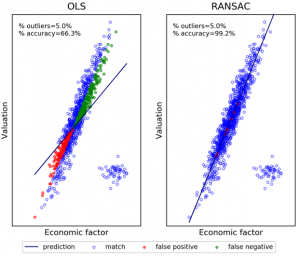# The seven reasons most econometric investments failMarcos Lopez de Prado, recently named 2019 Quant of the Year by the Journal of Portfolio Management, has released a presentation entitled The seven reasons most econometric investments fail.

Lopez de Prado’s overall point is that many widely used econometric approaches in finance either rely on misleading p-value statistics, or else rely on strong assumptions that are typically not satisfied by financial phenomena. Also, most econometric methods in finance do not pay sufficient attention to overfitting, either in the training set or in the testing set. These tools were, for the most part, developed in scientific fields such as biology and are not appropriate for investment and finance. As a result, he sadly concludes, “most econometric-based investment strategies are likely to be false.”

Here is a brief summary of his “seven reasons”:

1. Most econometric analyses typically rely on structured datasets, whereas the most interesting datasets are unstructured. In other words, “econometric models effectively model uninteresting data.”
2. Most econometric studies do not include methods to de-noise correlation matrices. As a result, “most econometric studies reach spurious conclusions, supported by noise, not signal.” Cross-sectional studies are particularly prone to classification errors — see figure.
3. Econometric specifications attempt to adjudicate the variance of a random variable in-sample, but in-sample adjudication is rarely useful for strategy development. In general, “regression is the wrong tool for investing.”
4. Many econometric methods require that the user simultaneously get the predictive variables and the functional form correct. Given the complexity of financial systems, these are unrealistic demands.
5. At a statistical level of p = 0.05, most strategies are false. Suppose, for instance, that the probability of a backtested strategy being profitable is 1%. Then at standard thresholds of 5% significance and 80% power, users are expected to make 58 discoveries, where 50 are false positives. In other words, 86% of the discoveries will be false. In practice, the percentage is even higher, often nearly 100%, due to multiple testing, specification errors and arbitrage forces.
6. Statistical models can be overfit in two ways: training set overfitting and testing set overfitting. The traditional econometric toolset fails to quantify, much less address these problems.
7. Many researchers employing traditional econometric tools and models fail to understand the extent to which overfitting compromises financial strategies. This is because even with Sharpe ratios of, say, three or higher, selection bias and confirmation bias errors can lead to false positives, often with financially disastrous outcomes.

For additional details, see Lopez de Prado’s The seven reasons most econometric investments fail.